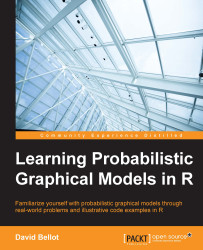•#### Learning Probabilistic Graphical Models in R#### Overview of this book

Probabilistic graphical models (PGM, also known as graphical models) are a marriage between probability theory and graph theory. Generally, PGMs use a graph-based representation. Two branches of graphical representations of distributions are commonly used, namely Bayesian networks and Markov networks. R has many packages to implement graphical models. We’ll start by showing you how to transform a classical statistical model into a modern PGM and then look at how to do exact inference in graphical models. Proceeding, we’ll introduce you to many modern R packages that will help you to perform inference on the models. We will then run a Bayesian linear regression and you’ll see the advantage of going probabilistic when you want to do prediction. Next, you’ll master using R packages and implementing its techniques. Finally, you’ll be presented with machine learning applications that have a direct impact in many fields. Here, we’ll cover clustering and the discovery of hidden information in big data, as well as two important methods, PCA and ICA, to reduce the size of big problems.
Learning Probabilistic Graphical Models in RCreditswww.PacktPub.comPrefaceFree Chapter
Probabilistic ReasoningExact InferenceLearning ParametersBayesian Modeling – Basic ModelsApproximate InferenceBayesian Modeling – Linear ModelsProbabilistic Mixture ModelsAppendixIndex## Learning with hidden variables – the EM algorithm

The last part of this chapter is an important algorithm that we will use again in the course of this book. It is a very general algorithm used to learn probabilistic models in which variables are hidden; that is, some of the variables are not observed. Models with hidden variables are sometimes called latent variable models. The EM algorithm is a solution to this kind of problem and goes very well with probabilistic graphical models.

Most of the time, when we want to learn the parameters of a model, we write an objective function, such as the likelihood function, and we aim at finding the parameters that maximize this function. Generally speaking, one could simply use a black-box numerical optimizer and just compute the relevant parameters given this function. However, in many cases, this would be intractable and too prone to numerical errors (due to the inherent approximations done by CPUs). Therefore it is generally not a good solution.

We...